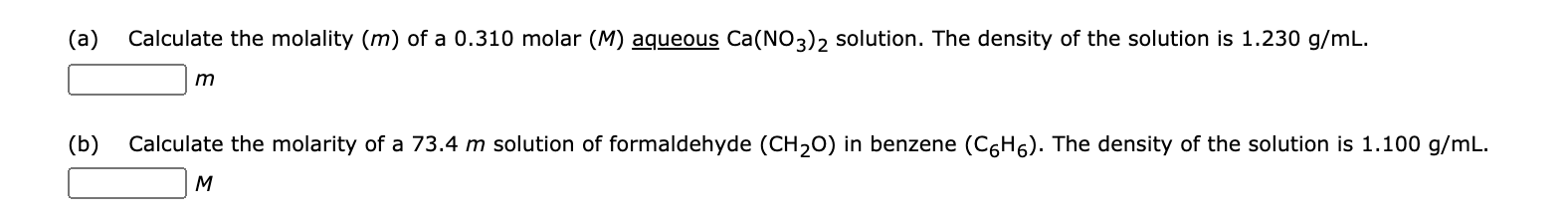# (a) Calculate the molality (m) of a 0.310 molar (M) aqueous Ca(NO3)2 solution. The density of the solution is 1.230 g/mL. m (b) Calculate the molarity of a 73.4 m solution of formaldehyde (CH20) in benzene (C6H6). The density of the solution is 1.100 g/mL. M

Questionhelp_outlineImage Transcriptionclose(a) Calculate the molality (m) of a 0.310 molar (M) aqueous Ca(NO3)2 solution. The density of the solution is 1.230 g/mL. m (b) Calculate the molarity of a 73.4 m solution of formaldehyde (CH20) in benzene (C6H6). The density of the solution is 1.100 g/mL. M fullscreen

1 Rating

### Want to see this answer and more?

Experts are waiting 24/7 to provide step-by-step solutions in as fast as 30 minutes!*

*Response times may vary by subject and question complexity. Median response time is 34 minutes for paid subscribers and may be longer for promotional offers.
Tagged in
Science
Chemistry

### Solutions